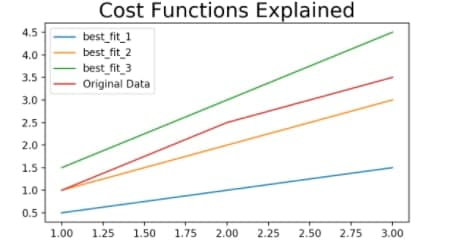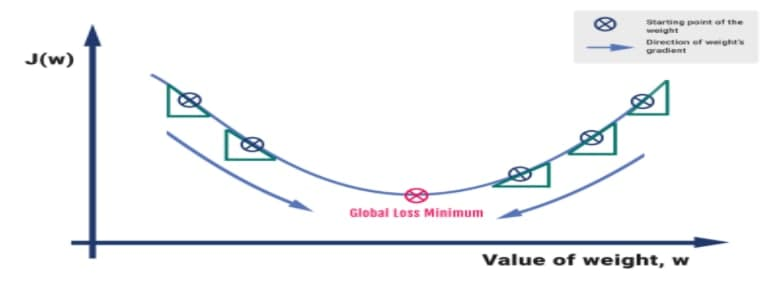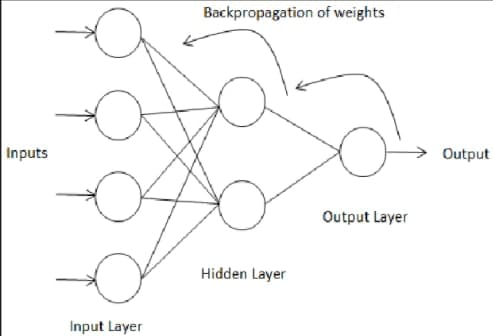When we build any neural network the main approach is to get as close to the actual output as we can but sometimes we don’t get the output as expected. To overcome this problem a cost function is defined which lets you know how much is the difference in residuals by analyzing the weights associated with them. Further that cost function acts as a feedback mechanism which then provides input to the hidden layer up to the input layer to make associated changes in their weights with the backpropagation method by fine tuning them.To know more about the working of neural networks please refer to my previous paper.

https://nm.dev/?post_type=sfwd-topic&p=28100&preview=true

In terms of the most popular algorithm for training neural networks, only gradient descent can live up to expectations. As a first-order optimization algorithm, gradient descent seeks a local minimum or maximum of a function iteratively. To minimize a cost/loss function (e.g. in a linear regression), this method is typically applied to machine learning  and deep learning.

These models learn over time through training data, and the cost function within gradient descent acts as a barometer, determining their accuracy with each parameter update iteration.

The Gradient descent is considered to be the first order optimization method since it takes the first derivative of the lost function.

The steps involved in the Neural Network are :

• We take the input equation:  Z = W0 + W1X1 + W2X2 + …+ WnXn and calculate the output, which is the predicted values of Y or the Ypred

• Calculate the error. It tells how much the model deviates from the actual observed values. It is always calculated as the Ypred – Yactual

In short we need to find the cost function which means the residual. The residual is the difference between the predicted outcome and the actual outcome.

The main target is to achieve the minimum error as we learned in machine learning.

Taking the computed loss value back to each layer updates the weights in a way that minimizes the loss. A loss function must have two qualities: it must be continuous and differentiable at each point.

When we started to compute the weights to train the network we came across too many permutations and combinations which took too much time. So to handle this problem gradient descent is considered to be the best amongst all since it starts computing based on the magnitude and direction of the flow of the network.

To this process we do a process that is called numerical gradient expressions which helps you to find out which way is downhill. Downhill is the term referred to the lowest point in the network finding which basically is our task since we want to minimize the cost functions.

The best way to solve this complex problem is by adapting the Gradient Descent algorithm.## When to apply

Once the output is generated it is not upto the mark so the next step is to apply the gradient descent algorithm with back propagation method to train the network properly according to the feedback received by the residual. Here it is how the backpropagation processes Back-propagation is just a way of propagating the total loss back into the neural network to know how much of the loss every node is responsible for, and subsequently updating the weights in such a way that minimizes the loss by giving the nodes with higher error rates lower weights and vice versa. Based on the partial derivatives of the loss function with respect to the parameters of the last layer, which do not influence over any other parameters of the network, the algorithm starts.## Where to process

It is applied to the output layer associated with the output with the help of back propagation to go back to the input layer by layer and by making the appropriate changes to the weights and biases of the perceptrons. So when the final changes have been made according to the received feedback then the network can start processing from the input layer to the output layer through a feed forward network process. If we could figure out which way was downhill so we can easily make changes in the weights which lead us to the downhill by decreasing the cost which will eventually solve the problem of time consuming computations. We need to keep the speed of convergence in mind for fast and optimum convergence. Below diagram shows a different range of convergence leads to different outcomes.## How to Integrate

The main goal now is to find out which way is downhill. We can find out this by obtaining the derivatives since here we are working on one one weight at a time hence the derivative would be partial. We can derive an expression for this derivative which can give us the rate of change of the J with respect to the weights with any value w. This will generalize the process as if the derivative is positive the lost function is going in the upward direction and if the partial derivative is negative it goes downhill which is exactly what we want. The sum of the squared residuals will give us the idea of how close and relatable our data is with respect to the input and by taking all the squared residual we can plot the actual data which will help the model to train and generate output.

This process will speed things up since we know which direction the cost function decreases and we can avoid the opposite direction to save time and effort. Now the question arises that how long do we need to carry out this process in order to get satisfied, so the answer is the moment when the cost function stops getting smaller. This is the method known as the Gradient Descent.

The gradient descent method is too impactful when the cost function is going in the same direction, but sometimes the direction of the cost function keeps changing which is also known as non convex. To deal with this problem we have Stochastic gradient descent but we will leave this topic for some other day.

## Limitations

There are some limitations of the gradient descent algorithms and are as follows:

Model network with a cost function which does not follow the same direction will lead to a serious problem since it might get stuck as it is only going into the downhill direction but that could also be the local minimum and our goal is to find the global minimum.

The other limitation on the list is that the learning rate does not change. This will lead us to solve the process by making the values constant which will synchronize all of it and leads to slow convergence. So to cope up with this situation what gradient descent does is that its convergence rate is faster with fast variables and slow to the slow variables which saves us lot of time and computational power.## Coding part

Lets move to the coding part and as you can see we have imported all the required libraries and initializing the program by loading and running it by declaring alpha values and maximum iterations.

				
%use s2 // always start your code with this magical words or program might not work
// some import statements

import java.awt.geom.Point2D// for performing operations on object ralation to 2D geometry
import java.util.ArrayList // insert arrays into programs
import java.util.function.DoubleUnaryOperator// operation on single double values operand that produce double valued result
import java.util.function.DoubleBinaryOperator// // operation on two double values operand that produce double valued result
import java.util.function.ToDoubleFunction// represent a function that produces a double value reuslt
import kotlin.jvm.JvmStatic// for generating static method if its a function


				
fun run() {
val alpha = 0.01
val maxIterations = 10000
val finalTheta = singleVarGradientDescent(data, 0.1, 0.1, alpha, maxIterations)
System.out.printf("theta0 = %f, theta1 = %f", finalTheta.x, finalTheta.y)
}




In this section we are launching the function and data types and initializing the iterations. We have taken theta value as 0.0 and processed the convergence to get the new theta in return.

Here the gradient theta is taken into consideration for double unary operations with respect to hypothesis.

This part of codes represents the theta by function hypothesis and than sigma is used to sum up all the multiple sums

Here we finally load the data and apply array list to return the data and at the end running it finally.

## Output## Conclusion

To conclude gradient descent i would like to say that it is the best optimizer that comes first into action when it comes to deal with lost function. Its main goal is to find the global minimum with minimum and accurate computation which eventually saves lots of time and avoids too much computational power.